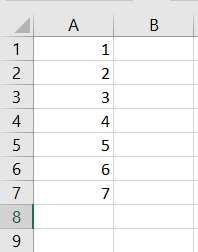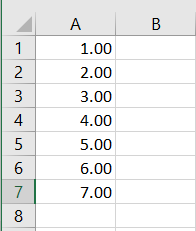# Convert Text to Number in Microsoft Excel VBA

Bilal Shahid Jan 30, 2023 Nov 21, 2022

VBA is a programming language for Microsoft Excel applications that provides users with various functions and features. In this article, we will learn about different methods to convert textual data to numeric form.

## Convert Text to Numbers in VBA

There can be a situation where you want to apply any arithmetic formula to Excel data but cannot do so.

This is because the numbers may be stored in text form! This article has the solution to this problem.

We know how to convert numbers to text simply by using the `CStr()` function. However, when we want to do the opposite, i.e., converting text to numbers form, we have multiple ways of doing so using VBA.

In this article, we will look at different conversion methods and a few examples to understand how these methods work.

## Method 1: Change `.NumberFormat` to `General`

In the first method, we select the cells by specifying the range and change the data format from `Text` to `General`, then set the values of the numbers.

Here is the generic code for it:

``````[X:Y].Select
With Selection
.NumberFormat = "General"
.Value = .Value
End With
``````

Here, `X:Y` represents the range of the cells. We have different number formats available in MS Excel, such as `General`, `Currency`, `Accounting`, `Text`, etc.

The `General` format keeps the number as it was entered.

For example, `22` will be kept as `22`, or `10.02` will be kept as `10.02`. The `.Value = .Value` statement is fetching and setting the cells’ values, which are now in the number format.

As a result, the selected range’s data will now be in numbers form, converted from the text. Let us see an example to further clarify the usage of this method.

Suppose we have the following sample data initially in our Excel sheet.Here is the code to convert the data in text to numeric form:

``````Sub method1()
[A1:A7].Select
With Selection
.NumberFormat = "General"
.Value = .Value
End With
End Sub
``````

Output:In this example, we had numbers `1-7` in text form. After running the above given VBA code, the data changed from text form to numbers form.

## Method 2: Use the `CSng()` Function

The second method can find all the numeric values in the sheet, even if the values are formatted as text. Then the `CSng()` function is used to convert this data into a single (common) data type.

Here is the generic code for this method:

``````For Each r In Sheets("SheetName").UsedRange.SpecialCells(xlCellTypeConstants)
If IsNumeric(r) Then
r.Value = CSng(r.Value)
r.NumberFormat = "0.00"
End If
Next
``````

Here, `Sheets("SheetName").UsedRange` selects all cells in use from the sheet. `SheetName` is the name of your Excel sheet.

The function `IsNumeric()` identifies any data that can be evaluated as a number. It is a Boolean type function and returns `True` or `False`.

The code checks each cell one by one, and if any data is detected that can be evaluated as a number, then its data type is first set to a single (common) datatype and then set to the `0.00` format. This means all the cells having data (and can be evaluated as numbers) now have a common data type with the format `0.00`.

Hence, the data has now been converted to numbers form. Let us see how the code works using an example.

We will use the same sample data as used in the previous example.Here is the code to convert text to numbers using the `CSng()` function:

``````Sub method2()
For Each r In Sheets("Sheet1").UsedRange.SpecialCells(xlCellTypeConstants)
If IsNumeric(r) Then
r.Value = CSng(r.Value)
r.NumberFormat = "0.00"
End If
Next
End Sub
``````

Output:In this example, the `IsNumeric()` function identifies all the cells in which data can be evaluated as numbers, and those cells (ranging from `A1` to `A7`) are now assigned a common type, i.e., format `0.00` or number form.

## Method 3: Multiply Cell Value by 1

This method is just a workaround and provides a shortcut trick to convert text to numeric form. The task you have to do in this method is simple: multiply each value in the cell by `1`.

This will convert the textual data to numeric form without changing the actual value of the data since multiplying with `1` does not bring any change numerically, and the data format will be changed implicitly to numbers due to a mathematical operation.

We have the following sample data:Here is the code to convert text to numbers by multiplying by 1:

``````Sub method4()
For Each r In Sheets("Sheet1").UsedRange.SpecialCells(xlCellTypeConstants)
If IsNumeric(r) Then
r.Value = r.Value * 1
End If
Next
End Sub
``````

## Conclusion

Conversion from one data type to another is handy, like converting numbers from text form to numbers form so we can apply arithmetical operations on the data.

In this article, we discussed different methods to convert `Text` to `Number`. We also discussed various examples to clarify our concepts and understand how the methods work.

We hope you were able to learn this concept. Keep learning!

Author: Bilal Shahid

Hello, I am Bilal, a research enthusiast who tends to break and make code from scratch. I dwell deep into the latest issues faced by the developer community and provide answers and different solutions. Apart from that, I am just another normal developer with a laptop, a mug of coffee, some biscuits and a thick spectacle!

GitHub